# Orbital mechanics and design¶

#### V. Hunter Adams (vha3), MAE 4160/5160, Spring 2020¶

In :
from IPython.display import Latex
from IPython.display import Image
from IPython.core.display import HTML


## In this document:¶

1. Review of Keplerian motion
2. Review of ellipse geometry and orbital parameters
3. Kepler's Laws (discussion and derivation)
4. Orbital perturbations
5. Geostationary orbits
6. Molniya orbits (frozen orbits and repeat ground tracks)
7. SSO orbits
8. Introduction to constellations
9. GPS constellatin
10. Iridium constellation

This lecture is about the orbits into which we place spacecraft. The next lecture is about how we get the spacecraft to those orbits.

## Orbit Mechanics Review¶

### Keplerian motion¶

We'll start by considering the two-body problem. This problem considers the interaction between two point masses that are only acted upon by the gravitational force (no perturbations), as shown below.

In :
Image(filename = "bodies.png", width=600, height=600)

Out: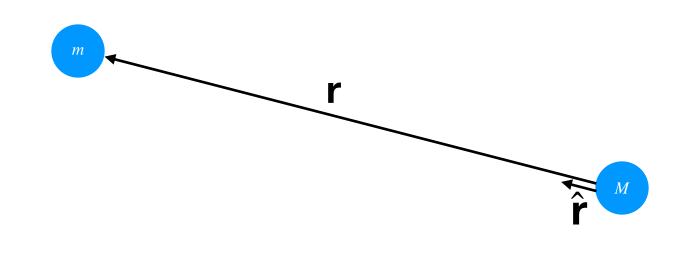$\textbf{r}$ is the vector separating $M$ and $m$ (points from $M$ to $m$). The unit vector that points in the direction of $\textbf{r}$ is $\hat{\textbf{r}}$. The forces acting on each body are given below:

\begin{align} \textbf{F}_m &= -G \frac{M m}{||\textbf{r}||^2} \hat{\textbf{r}}\\ \textbf{F}_M &= G \frac{M m}{||\textbf{r}||^2} \hat{\textbf{r}} \end{align}

Where $G$ is Newton's Gravitational Constant. In order to find the equation of motion for $m$, we apply Newton's second law:

\begin{align} m \ddot{\textbf{r}} &= -G \frac{Mm}{||\textbf{r}||^2} \hat{\textbf{r}} \end{align}

Simplifying:

\begin{align} \boxed{\ddot{\textbf{r}} = -G \frac{M}{||\textbf{r}||^2} \hat{\textbf{r}}} \end{align}

We often see this equation written in terms of $\mu = GM$:

\begin{align} \ddot{\textbf{r}} = -\frac{\mu}{||\textbf{r}||^2} \hat{\textbf{r}} \end{align}

This equation describes planar motion, which is characterized by Kepler's Laws. We'll discuss those momentarily. Before doing so, I'd like to make an observation. Through the notation used above, we've implied that one of the masses, $M$, is significantly larger than the other mass, $m$. One represents a planet, the other a spacecraft. We can define a polar coordinate system with its origin at the center of mass of the more massive body, $M$, and parametrize the vector $\textbf{r}$ in terms of those polar coordinates, as shown below:

\begin{align} \textbf{r} &= \rho \hat{\rho} + \theta \hat{\theta} \end{align}

Where $\rho$ is the distance separating the two bodies, and $\theta$ is the polar angle that rotates that magnitude vector to the position of the mass (true anomaly). The above equation of motion for the vector $\textbf{r}$ can be separated into an equation of motion for the radial component $\rho$ and the angular component $\theta$ as shown below:

\begin{align} \boxed{\ddot{\rho}= -\frac{G M}{\rho ^2} + \rho\dot{\theta}^2} \end{align}
\begin{align} \boxed{\ddot{\theta} = -\frac{2\dot{\theta}\dot{\rho}}{\rho}} \end{align}

These equations are useful for discussing Kepler's Laws.

### Kepler's First Law¶

#### Discussion¶

Kepler's first law states that orbits are conic sections (circles, ellipses, parabolas, and hyperbolas). The solution to the above two-body problem is given below. Any conic section can be a solution to this equation.

\begin{align} \rho(\theta) &= \frac{a(1-e^2)}{1+e\cos{\theta}}\\ \end{align}

Where $a$ is the semimajor axis, $e$ is the eccentricity, and $\theta$ is the true anomaly. It's worth pausing for a moment to review some ellipse geometry and orbital mechanics vocabulary.

###### A brief aside on ellipse geometry¶

A labeled picture:

In :
Image(filename = "ellipse.png", width=600, height=600)

Out: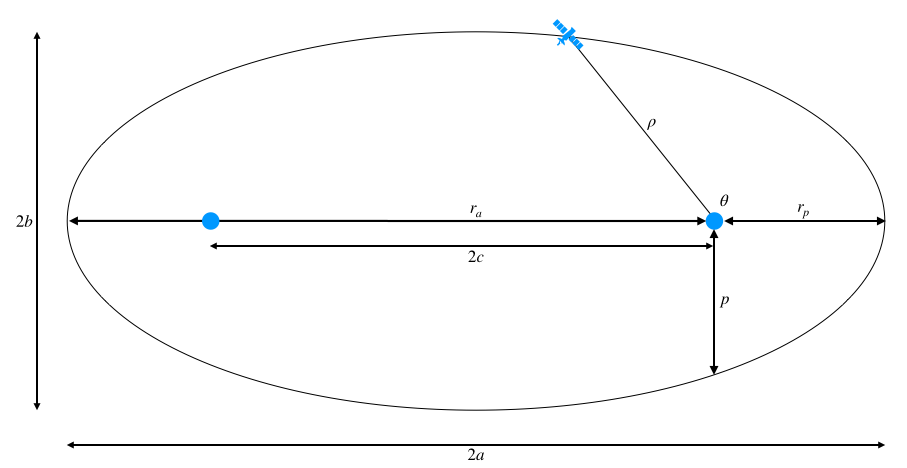Some definitions:

\begin{align} a&: \text{ semi-major axis}\\ b&: \text{ semi-minor axis}\\ e&: \text{ eccentricity}\\ \theta&: \text{ true anomaly}\\ \rho &: \text{ radial distance (polar coords)}\\ p&: \text{ semi-latus rectum}\\ c&: \text{ linear eccentricity}\\ r_a&: \text{ apoapsis distance}\\ r_p&: \text{ periapsis distance}\\ A&: \text{ area} \end{align}

Some useful relationships:

\begin{align} 1 &= \frac{x^2}{a^2} + \frac{y^2}{b^2}\\ A &= \pi a b\\ r_p &= a(1-e)\\ r_a &= a(1+e)\\ e &= \frac{c}{a} = \sqrt{1 - \frac{b^2}{a^2}} = \frac{r_p - r_a}{r_p + r_a}\\ p &= \frac{h^2}{\mu} = a(1-e^2)\\ \rho &= \frac{a(1-e^2)}{1+e\cos{\theta}} = \frac{r_p(1-e)}{1+e\cos{\theta}} = \frac{p}{1 + e\cos{\theta}} \end{align}
###### A reminder about orbital parameters¶

These are the six parameters that are used to uniquely specify an orbit. They divide into three categories: those which define the shape of the orbit, those which define the orientation of the orbit in inertial space, and those which define the location of the spacecraft on that orbit.

Size and shape of orbit: The following two orbital parameters define the size and shape of an orbit:

1. Semi-major axis, $a$
2. Eccentricity, $e$

Orientation of the orbit: The following three orbital parameters define the orientation of the orbit in space:

1. Inclination, $i$
2. Longitude of the ascending node, $\Omega$
3. Argument of periapsis, $\omega$

Location of satellite in orbit: The remaining parameter specifies the location of the spacecraft along the orbit.

1. True anomaly, $\theta$ ($\nu$ in the illustration below)

See the illustration below.

In :
Image(filename = "params.png", width=600, height=600)

Out: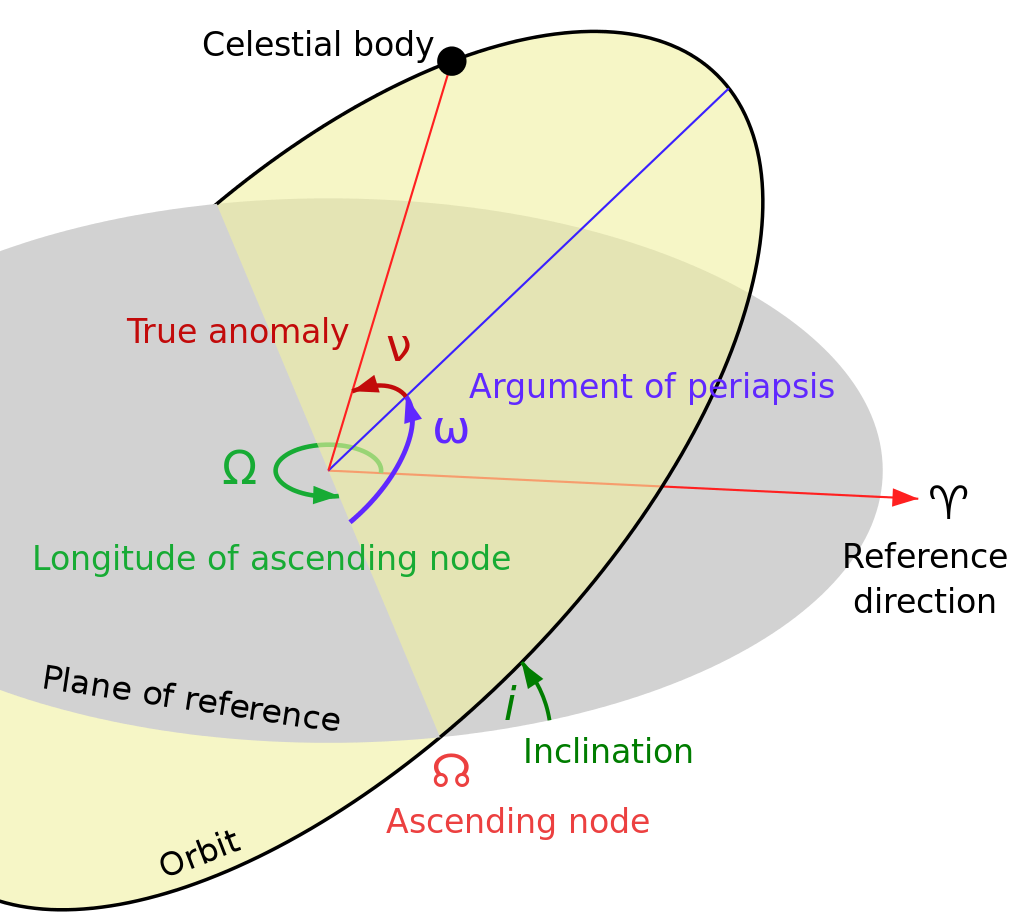#### Derivation¶

Consider the radial equation of motion for the spacecraft in the xy plane, provided above:

\begin{align} \ddot{\rho} - \rho\dot{\theta}^2= -\frac{G M}{\rho ^2} \end{align}

This can be rewritten in terms of the angular momentum, $h = m\rho\dot{\theta}$:

\begin{align} \ddot{\rho} - \frac{h^2}{m^2 \rho^3} &= -\frac{GM}{\rho^2} \end{align}

In the previous section, it was shown that $h$ is constant. Pulling a trick, make the following substitution:

\begin{align} \rho(\theta) &= u^{-1}(\theta) \end{align}

This implies the following:

\begin{align} \dot{\rho} &= -\frac{\dot{u}}{u^2}\\ &= -\rho^2(\theta)\frac{du}{d\theta}\frac{d\theta}{dt}\\ &= -\rho^2(\theta)\dot{\theta}\frac{du}{d\theta}\\ &= -h \frac{du}{d\theta} \end{align}
\begin{align} \ddot{\rho} &= -h \frac{d^2u}{d\theta^2}\frac{d\theta}{dt}\\ &= -h\dot{\theta}\frac{d^2u}{d\theta^2}\\ &= -u^2h^2\frac{d^2u}{d\theta^2} \end{align}

This allows the radial equation of motion to be rewritten in linear form:

\begin{align} \frac{d^2u}{d\theta^2} + u &= \frac{GM}{h^2} \end{align}

This differential equation has a general solution given by:

\begin{align} u(\theta) &= \frac{GM}{h^2}\left[1+e\cos{(\theta-\theta_0)}\right] \end{align}

for arbitrary constants $e$ and $\theta_0$. Without loss of generality, we can set $\theta_0$ to zero and solve for $\rho(\theta)$:

\begin{align} \rho(\theta) &= \frac{\rho_c}{1+e\cos{\theta}} \end{align}

where $\rho_c = \frac{h^2}{GM}$. This can be recognized as the equation for an ellipse. More commonly, $\rho_c$ is written in terms of the semimajor axis and eccentricity of the ellipse:

\begin{align} \rho(\theta) &= \frac{a(1-e^2)}{1+e\cos{\theta}} \end{align}

### Kepler's Second Law¶

#### Discussion¶

Kepler's second law states that the line segment which joins a satellite and the central body sweeps equal areas in equal times. This is tied to the conservation of angular momentum, as shown below.

#### Derivation¶

Taking a step back, let us consider the angular momentum for the spacecraft, modeled as a point mass:

\begin{align} {\bf{h}} &= I\dot{\theta}\\ &= m\rho^2\dot{\theta} \end{align}

Multiply both sides of the equation for tangential motion (provided above) by $m\rho$:

\begin{align} m\rho^2\ddot{\theta} + 2m\rho\dot{\theta}\dot{\rho} = 0 \end{align}

The above equation could be rewritten as:

\begin{align} \frac{d}{dt}\left(m\rho^2\dot{\theta}\right) &= 0\\ \frac{d}{dt}{\bf{h}} &= 0\\ \end{align}

This shows that angular momentum is conserved under our dynamics assumptions. Consider the path that the spacecraft takes in a very short amount of time, shown below:

In :
Image(filename = "kepler.png", width=600, height=600)

Out: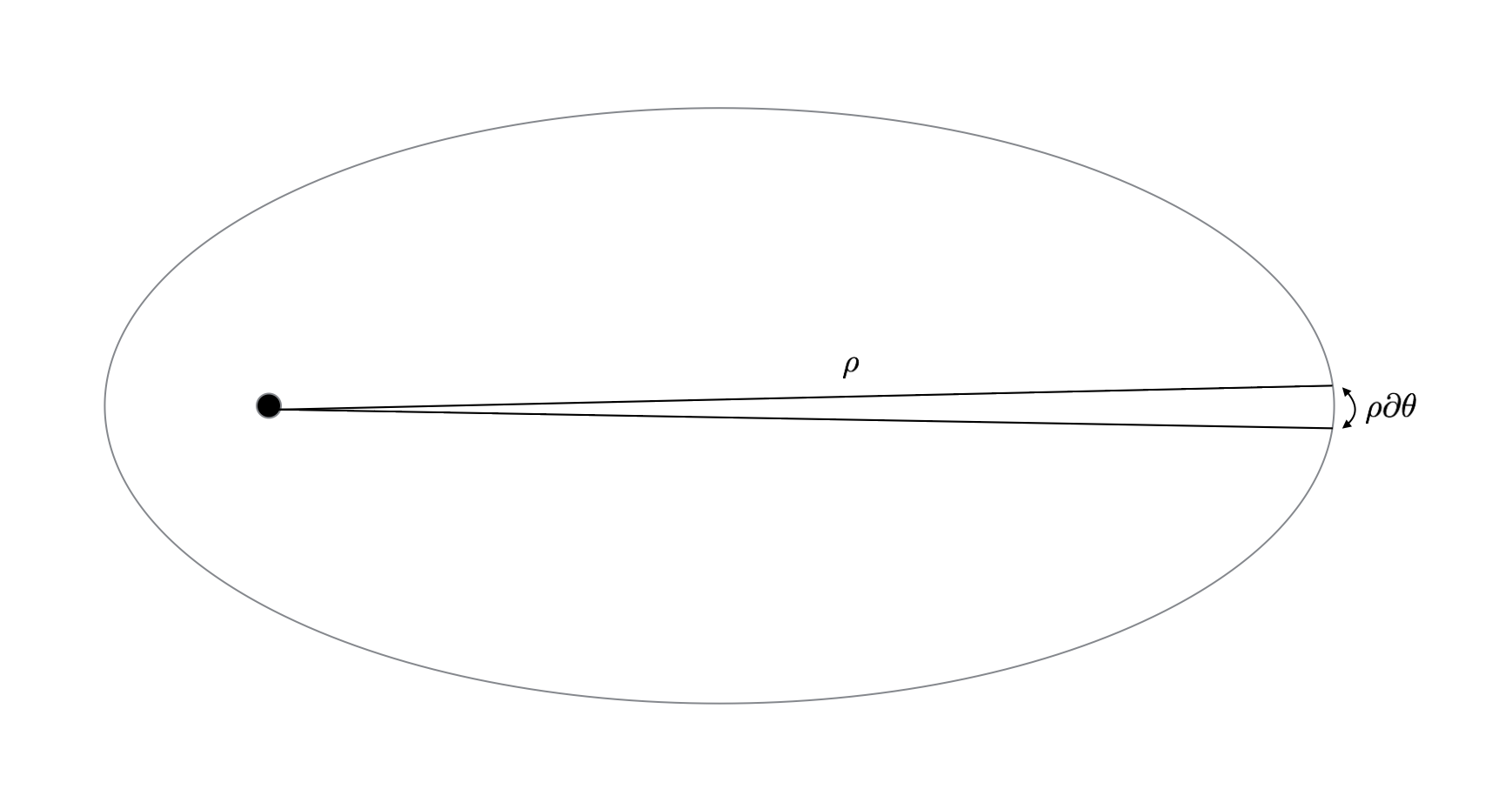The area swept out by the spacecraft is approximately a triangle with height $\rho$ and base $\rho \partial{\theta}$. The area of this triangle is given by:

\begin{align} A &= \frac{1}{2}\rho^2\partial{\theta} \end{align}

The rate at which this area is swept out is given by:

\begin{align} \frac{dA}{dt} &= \frac{1}{2}\rho^2 \frac{d\theta}{dt}\\ &= \frac{1}{2}\rho^2 \dot{\theta}\\ &= \frac{{\bf{h}}}{2m} \end{align}

It has been shown that the angular momentum is constant, so the rate of area accumulation is also constant. The spacecraft sweeps out equal areas in equal times.

### Kepler's Third Law¶

#### Discussion¶

Kepler's third law states that the square of the orbital period is proportional to the cube of the semi-major axis of the orbit. Specifically:

\begin{align} T^2 &= \frac{4\pi^2a^3}{GM} \end{align}

#### Derivation¶

It has been shown that the trajectory of a spacecraft under the dynamics assumptions traces out an ellipse, and that the spacecraft sweeps equal areas in equal times. This can be used to find the relationship between the semimajor axis of the ellipse and the orbital period of the spacecraft.

\begin{align} T &= \frac{A}{dA/dt} \end{align}

$A$ is the area of the ellipse, given by $\pi ab$. $dA/dt$ is, as shown above, $\frac{h}{2m}$. Thus:

\begin{align} T &= \frac{2m\pi ab}{h} \end{align}

From the equations for conic sections:

\begin{align} a &= \frac{\rho_c}{1-e^2}\\ b &= \frac{\rho_c}{\sqrt{1-e^2}}\\ \rho_c &= \frac{h^2}{GM} \end{align}

Thus:

\begin{align} T^2 &= \frac{4\pi^2a^3}{GM} \end{align}

The square of the orbital period is proportional to the cube of the semimajor axis.

In reality, the orbits of spacecraft are not perfectly described by Kepler's equations. This is because there exist perturbations which cause additional accelerations on the spacecraft. These perturbations come in the form of distortions to the point-mass gravitational potential that Kepler's equations assume (which then manifest as accelerations), and in the form of external forces acting on the spacecraft. The relative magnitudes of these accelerations change with altitude, as shown below. Incidentally, they also change in interesting ways with the size of the spacecraft. A centimeter or millimeter-scale spacecraft experiences perturbations that are quite different from those of large spacecraft. See Justin Atchison's thesis.

In :
Image(filename = "perts.png", width=600, height=600)

Out: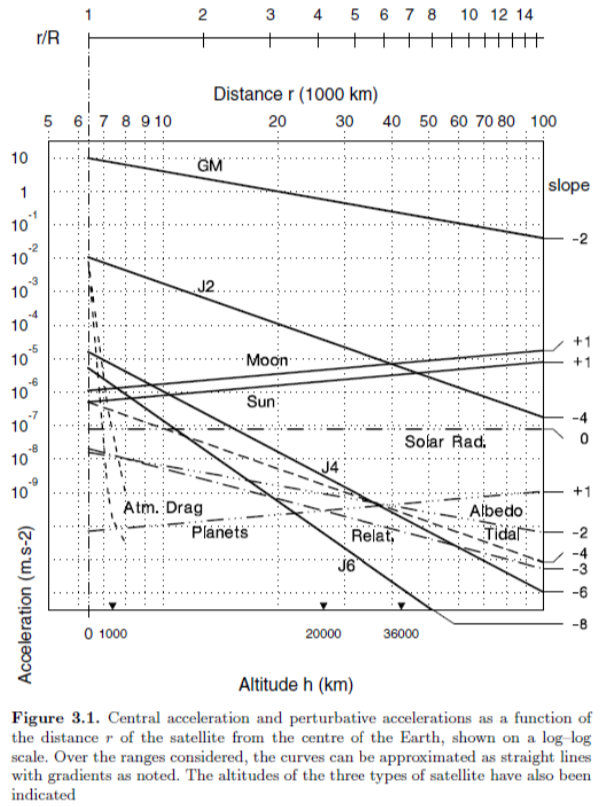#### J2 Perturbations¶

The above plot shows that perturbations from $J2$ are, by far, the dominant source of perturbation for spacecraft orbiting between ~400 km and Geostationary orbit. $J2$ comes from the oblateness of the Earth.

The Keplerian equations from the previous section make the assumption that the Earth's gravitational potential is given by the following equation:

\begin{align} U = -\frac{GMm}{\rho} \end{align}

This would be true if the Earth were a perfect, homogenous sphere. In reality, the Earth is neither spherical nor perfectly homogenous in density. It is lumpy. The actual gravitational sphere is approximated using spherical harmonics, shown below.

In :
Image(filename = "model.png", width=600, height=600)

Out: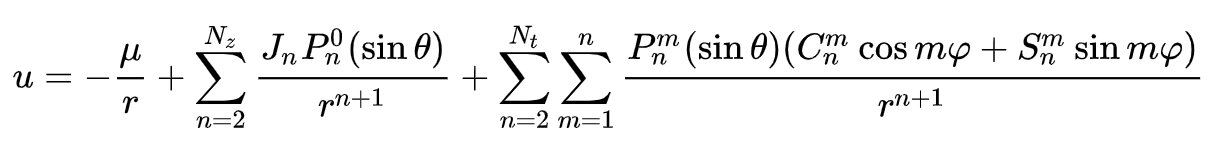The first term in this expression should look familiar. This is the point-mass potential. The first summation is known as the zonal terms, and the last summation is known as the tesseral terms. You can see that each of the zonal terms has a term $J_n$ in the summation, and that the summation starts at 2. The $J2$ perturbations are those caused by the first element of this summation. This is the element which describes equatorial oblateness (an Earth which is fat at the equator). Higher-order terms describe more complex mass distributions and, as shown in the plot above, are less significant.

The effects of Earth's oblateness can be determined by adding the $J2$ term to the potential and solving using Lagrange's equations. The effects on the orbital parameters are given below.

\begin{align} \dot{\Omega} &= -\frac{3}{2(1-e^2)^2}nJ_2\left(\frac{R_E}{a}\right)^2 \cos{i}\\ \dot{\omega} &= \frac{3}{4(1-e^2)^2}nJ_2\left(\frac{R_e}{a}\right)^2\left(5\cos^2{i} - 1\right) \end{align}

In addition to these two orbital parameters, $J2$ also affects the mean motion:

\begin{align} \Delta n &= \frac{3}{4(1-e^2)^{\frac{3}{2}}} n J_2 \left(\frac{R_E}{a}\right)^2 \left(3\cos^2{i} - 1\right) \end{align}

These effects can be summarized as follows:

1. The orbital plane rotates (since $\dot{\Omega} \neq 0$). This is called nodal precession and is very important for sun-synchronous orbits, as we'll discuss momentarily.
2. The argument of perigee rotates within the orbital plane. This is called apsidal precession and is very important for frozen orbits (see Molniya).
3. The mean motion changes. It may be faster or slower than Keplerian depending on inclination, as shown in the equation above.

#### Atmospheric Drag¶

Below a certain altitude, atmospheric drag begins to dominate. The acceleration due to drag is modeled as:

\begin{align} a_d(r,V) &= -\frac{1}{2} \rho(r) V(r)^2 \cdot \frac{C_DA}{m} \end{align}

where $\rho(r)$ is the atmospheric density at position $r$, and $V(r)$ is the velocity of the spacecraft at position $r$. $C_D$ is the drag coefficient, $A$ the effective surface area, and $m$ the mass.

Question: For elliptical orbits, where do you expect for drag to be the most significant?

## Orbit Design¶

### Geostationary¶

The Cool Idea: Let's provide persistent, high-definition television coverage to the United States.

The Quantified Requirement: We require persistent transmission of [frequency range] radio waves to [lat/lon range] at [bandwidth]. (Not quite specific enough, but good enough for our conversation).

That's a hard problem. If you want your transmission to be received by everyone in the United States, you're either going to need a lot of transmitters, or you're going to need something high enough to see the whole geographic area that you're interested in. So, we're in the world now of spaceflight mechanics. We're going to use satellites to provide persistent coverage for this particular geographic area. Because satellites are expensive, we also want to do this with the minimum possible number of satellites.

So, this is a dead-simple example. What orbit would you design for this particular mission?

The answer: Geostationary orbit. We can park a satellite in geostationary orbit with a view of the United States and, for all time, it will just stay there happily transmitting to the United States.

If we look at a map of some of the satellites that occupy this orbit, you'll see that many of them are there to do things like we just talked about. I was specifically thinking of DirecTV in this example, which you can see here. If you go to this Wikipedia page: https://en.wikipedia.org/wiki/List_of_satellites_in_geosynchronous_orbit, you'll see that all the satellites in geosync have one of the following purposes:

Image("Geostationary.png", width=800)